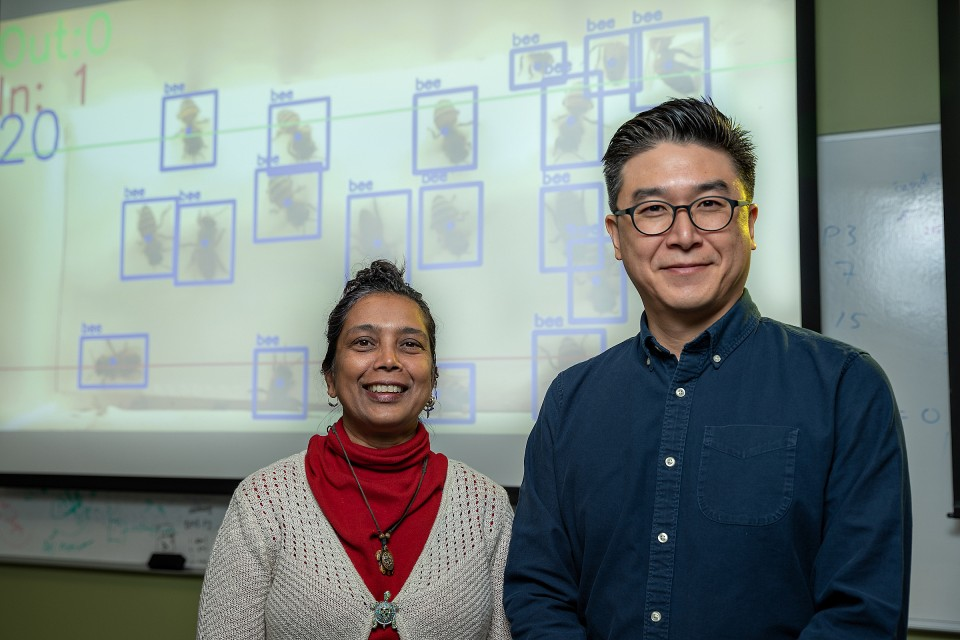## Computer Science Faculty Publications and Presentations

Article

2023

#### Abstract

We study the classical scheduling problem on parallel machines %with precedence constraints where the precedence graph has the bounded depth h. Our goal is to minimize the maximum completion time. We focus on developing approximation algorithms that use only sublinear space or sublinear time. We develop the first one-pass streaming approximation schemes using sublinear space when all jobs' processing times differ no more than a constant factor c and the number of machines m is at most 2nϵ3hc. This is so far the best approximation we can have in terms of m, since no polynomial time approximation better than 43 exists when m=n3 unless P=NP. %the problem cannot be approximated within a factor of 43 when m=n3 even if all jobs have equal processing time. The algorithms are then extended to the more general problem where the largest αn jobs have no more than c factor difference. % for some constant 0